Next: SYLVESTER'S MATRIX THEOREM Up: Matrices and multichannel time Previous: Matrices and multichannel time

# REVIEW OF MATRICES

A set of simultaneous equations may be written as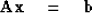(1)
where A is a square matrix (nonsquare matrices are taken up in Chap. 6 on least squares) and x and b are column vectors. In acase, (1) becomes(2)
Equation (2) has a simple geometric interpretation. The two columns of the matrix and the column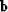are regarded as vectors in a plane. Equation (2) says x1 times the first column vector plus x2 times the second column vector equals thecolumn vector. Difficulty arises when the two column vectors of the matrix point in the same direction. Unlessjust happens to be that direction, no solution x1, x2 is possible. The same thing may be said about the general case. A solution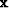to equation (1) exists iflies in the space spanned by the columns of.In most practical situations the matrixand the columnarise from independent considerations so that it is often reasonable to require the columns ofto span a space which will contain an arbitraryvector. Ifis an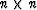matrix, then its columns are required to span an n-dimensional space. In particular, the n-dimensional parallelopiped with edges given by the columns ofshould not have a zero volume. Such a volume is given by the determinant of.

Another set of simultaneous equations which arises frequently in practice is the so-called homogeneous equations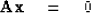(3)
This set always has the solutionwhich is often called the trivial solution. For (3) to have nontrivial solution values forthe determinant ofshould vanish, meaning that the columns ofdo not span an n-dimensional space. We will return later to the subject of actually solving sets of simultaneous equations.

This set always has the solutionwhich is often called the trivial solution. For (3) to have nontrivial solution values forthe determinant ofshould vanish, meaning that the columns ofdo not span an n-dimensional space. We will return later to the subject of actually solving sets of simultaneous equations.

A most useful feature of matrices is that their elements may be not only numbers but that they may be other matrices. Viewed differently, a big matrix may be partitioned into smaller submatrices. A surprising thing is that the product of two matrices is the same whether there are partitions or not. Study the identity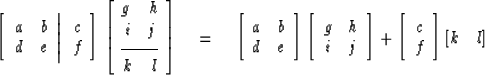(4)
In terms of summation notation, the left-hand side of (4) means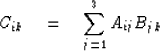(5)
whereas the right-hand side means(6)
Equations (5) and (6) are obviously the same; this shows that this partitioning of a matrix product is merely rearranging the terms. Partitioning does not really do anything at all from a mathematical point of view, but it is extremely important from the point of view of computation or discussion.

We now utilize matrix partitioning to develop the bordering method of matrix inversion. The bordering method is not the fastest or the most accurate method but it is quite simple, even for nonsymmetric complex-valued matrices, and it also gives the determinant and works for homogeneous equations. The bordering method proceeds by recursion. Given the inverse to amatrix, the method shows how to find the inverse of a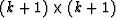matrix, which is the same oldmatrix with an additional row and column attached to its borders. Specifically,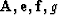, andare taken to be known in (7). The task is to find, and z.(7)

The first thing to do is multiply the partitions in (7) together. For the first column of the product we obtain(8) (9)
A choice of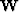of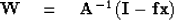(10)
leads to (8) being satisfied identically. This leaves x still unknown, but we may find it by substituting (10) into (9)(11)
Now, to get the column unknowns y and z, we compute the second column of the product (7)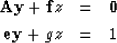(12) (13)
Multiply (12) by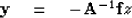(14)
This gives the column vector y within a scale factor z. To get the scale factor, we insert (14) into (13)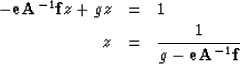(15)
It may, in fact, be shown that the determinant of the matrix being inverted is given by the product over all the bordering steps of the denominator of (15). Thus, if at any time during the recursion the denominator of (15) goes to zero, the matrix is singular and the calculation cannot proceed.

Let us summarize the recursion:   One begins with the upper left-hand corner of a matrix. The corner is a scalar and its inverse is trivial. Then it is considered to be bordered by a row and a column as shown in (7). Next, we find the inverse of thismatrix. The process is continued as long as one likes. A typical step is first compute z by (15) and then computeof one larger size by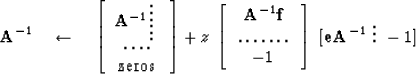(16)
where (16) was made up from (10), (11), and (14). A Fortran computer program for matrix inversion based on the bordering method is shown below:

                SUBROUTINE CMAINE (N,B,A)
C	A=MATRIX INVERSE OF B
COMPLEX B,A,C,R,DEL
DIMENSION  A(N,N),B(N,N),R(100),C(100)
DO 10 I=1,N
DO 10 J=1,N
10  A(I,J)=0
DO 40 L=1,N
DEL=B(L,L)
DO 30 I=1,L
C(I)=0.
R(I)=0.
DO 20 J=1,L
C(I)=C(I)+A(I,J)*B(J,L)
20  R(I)=R(I)+B(L,J)*C(I)
30  DEL=DEL-B(L,I)*C(I)
C(L)=-1.
R(L)=-1.
DO 40 I=1,L
C(I)=C(I)/DEL
DO 40 J=1,L
40  A(I,J)=A(I,J)+C(I)*R(J)
RETURN
END


It is instructive to see what becomes ofif A is perturbed steadily in such a way that the determinant of A becomes singular. If the element g in the matrix of (7) is moved closer and closer to,then we see from (15) that z tends to infinity. What is interesting is that the second term in (16) comes to dominate the first, and the inverse tends to infinity times the product of a columnwith a row r.

The usual expressionsor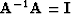in the limit of small z-1 tend to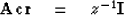(17)
or(18)
In the usual case (rank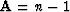, not rank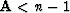) where neither c nor r vanish identically, (17) and (18) in the limit z-1 = 0 become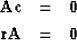(19) (20)

In summary, then, to solve an ordinary set of simultaneous equations like (1), one may compute the matrix inverse of A by the bordering method and then multiply (1) byobtaining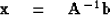(21)
In the event b vanishes, we are seeking the solution to homogeneous equations and we expect that z will explode in the last step of the bordering process. (If it happens earlier, one should be able to rearrange things.) The solution is then given by the column c in (19).

The row homogeneous equations of (20) was introduced because such a set arises naturally for the solution to the row eigenvectors of a nonsymmetric matrix. In the next section, we will go into some detailed properties of eigenvectors. A column eigenvector c of a matrix A is defined by the solution to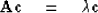(22)
whereis the so-called eigenvalue. At the same time, one also considers a row eigenvector equation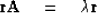(23)
To have a solution for (22) or (23), one must have det.After finding the roots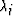of the polynomial det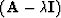,one may form a new matrix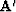for eachby(24)
then the solution to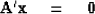(25)
arises from the column c at the last step of the bordering. It is the column eigenvector. Likewise, the row eigenvector is the row in the last step of the bordering algorithm.

## EXERCISES:

1. Indicate the sizes of all the matrices in equations (8) to (15).
2. Show how (16) follows from (10), (11), (14), and (15).Next: SYLVESTER'S MATRIX THEOREM Up: Matrices and multichannel time Previous: Matrices and multichannel time
Stanford Exploration Project
10/30/1997